# IB DP Physics: SL复习笔记2.4.4 Collisions & Explosions

### Collisions & Explosions

• In both collisions and explosions, momentum is always conserved
• However, kinetic energy might not always be
• A collision (or explosion) is either:
• Elastic – if the kinetic energy is conserved
• Inelastic – if the kinetic energy is not conserved
• Collisions are when objects strike against each other
• Elastic collisions are commonly those where objects colliding do not stick together and then move in opposite directions
• Inelastic collisions are commonly those where objects collide and stick together after the collision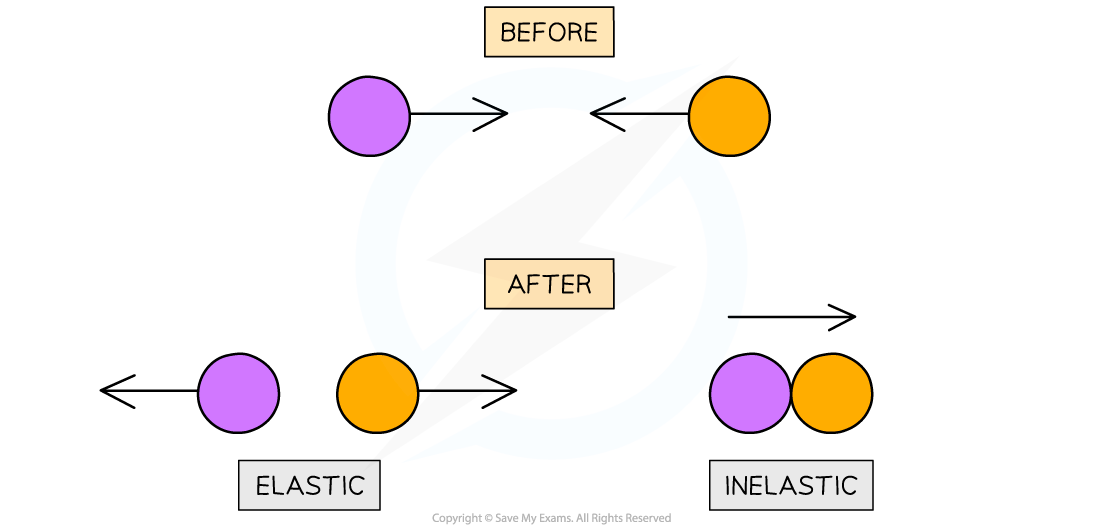Elastic collisions are where two objects move in opposite directions. Inelastic collisions are where two objects stick together

• An explosion is commonly to do with recoil
• For example, a gun recoiling after shooting a bullet or an unstable nucleus emitting an alpha particle and a daughter nucleus
• To find out whether a collision is elastic or inelastic, compare the kinetic energy before and after the collision
• The equation for kinetic energy is:#### Worked Example

Two similar spheres, each of mass m and velocity v are travelling towards each other. The spheres have a head-on elastic collision.What is the total kinetic energy after the impact?#### Worked Example

Trolley A of mass 0.80 kg collides head-on with stationary trolley B at speed 3.0 m s–1. Trolley B has twice the mass of trolley A.The trolleys stick together and travel at a velocity of 1.0 m s–1.Determine whether this is an elastic or inelastic collision.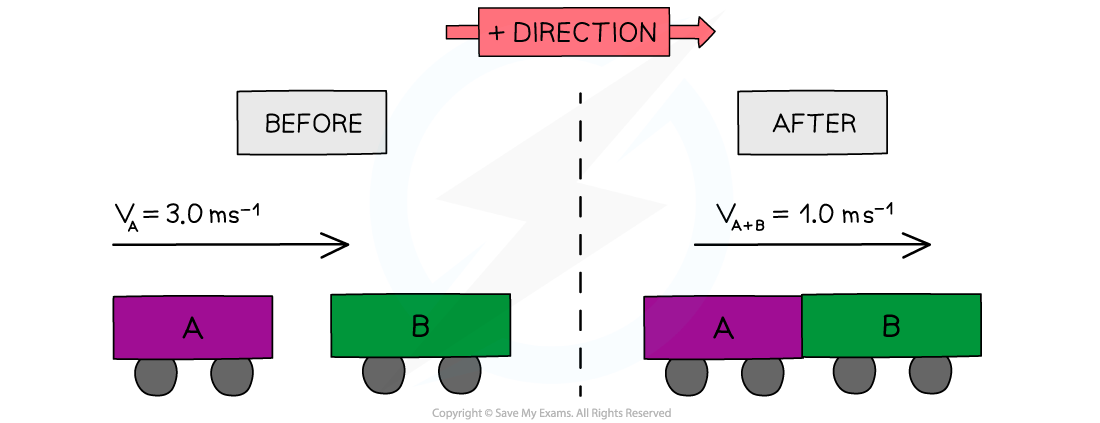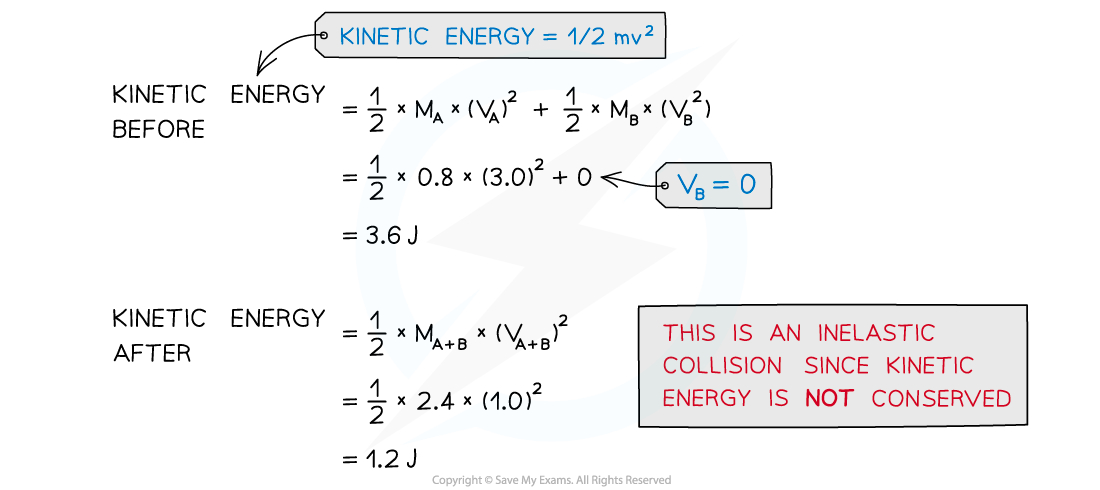#### Exam Tip

If an object is stationary or at rest, its velocity equals 0, therefore, the momentum and kinetic energy are also equal to 0When a collision occurs in which two objects are stuck together, treat the final object as a single object with a mass equal to the sum of the two individual objectsDespite velocity being a vector, kinetic energy is a scalar quantity and therefore will never include a minus sign - this is because in the kinetic energy formula, mass is scalar and the v2 will always give a positive value whether its a negative or positive velocity

### Applying Conservation of Momentum

• The principle of conservation of momentum can be used to solve various types of problems for isolated systems such as problems involving:
• Collisions
• Fluid jets
• Conveyor belts
• Explosions & many more
• Kinetic energy can also be used to check if a collision is elastic or inelastic
• In elastic collisions, momentum and kinetic energy are conserved
• In inelastic collisions, momentum is conserved, but kinetic energy is not
• Inelastic includes explosions

#### Worked Example

Two trolleys X and Y are of equal mass. Trolley X moves towards trolley Y which is initially stationary. After the collision, the trolleys join and move off together.Show that this collision is inelastic.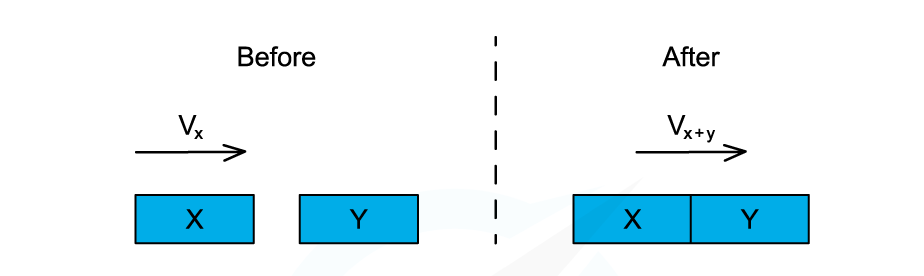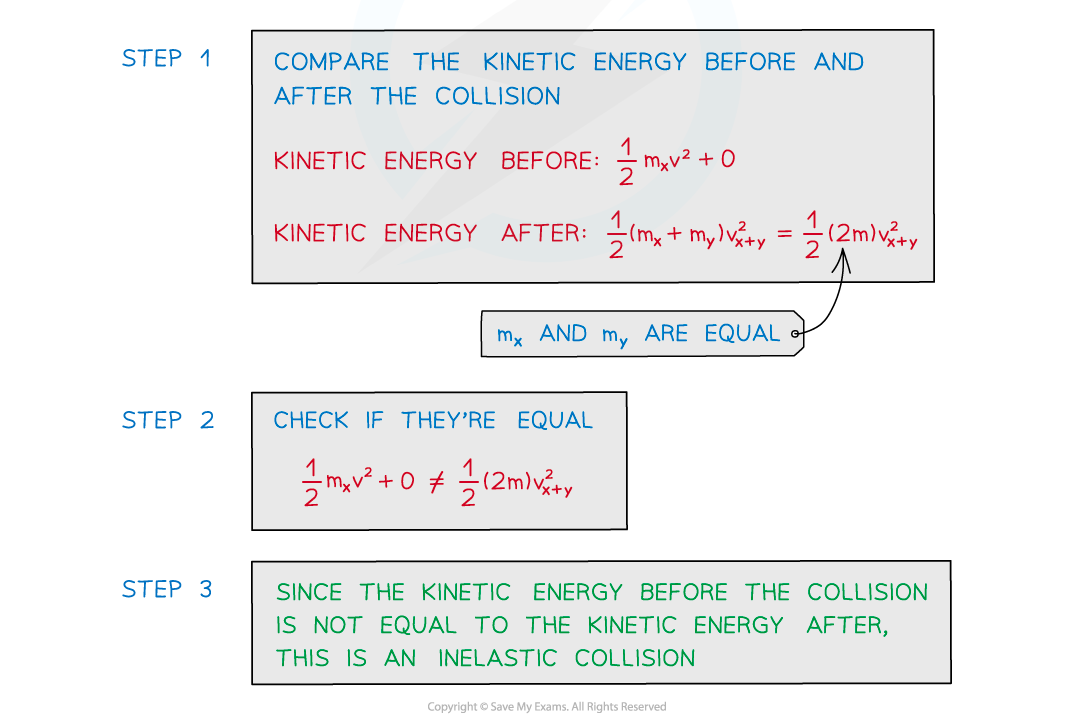#### Worked Example

A 2 kg crossbow is fired and a 100 g arrow is fired horizontally. The arrow is released from the crossbow at 40 m s−1.Determine the magnitude of the recoil velocity of the crossbow.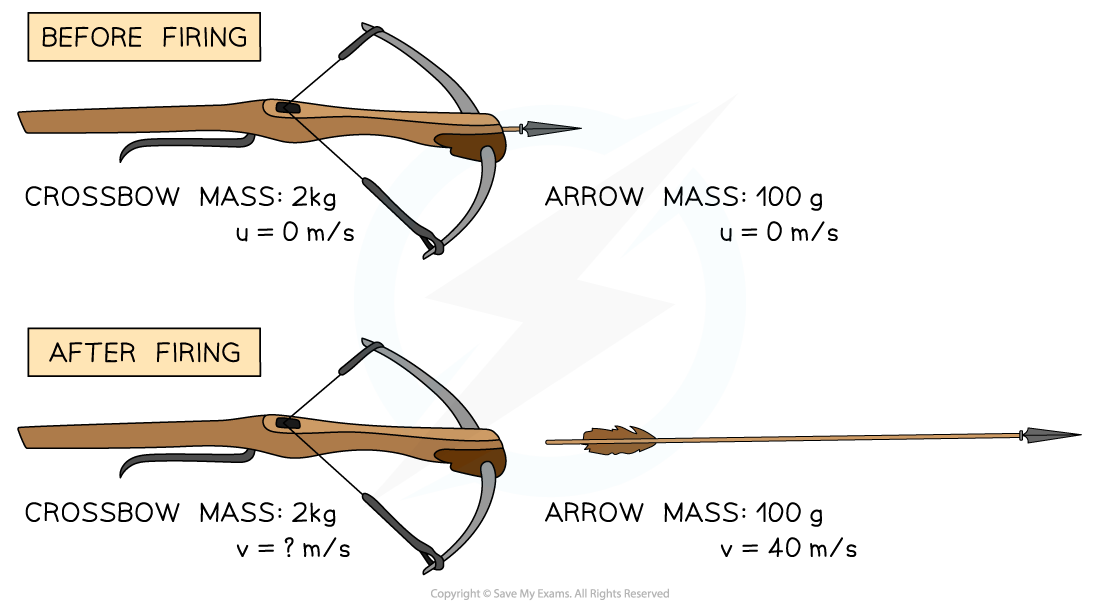Step 1: List the known quantities

• Mass of the crossbow: 2 kg
• Mass of the arrow: 100 g = 0.1 kg
• Speed of arrow during release: 40 m s−1

Step 2: Determine the momentum before release

• Since before release neither the arrow nor the crossbow was moving, their momentum together is 0 kg m s−1

Step 3: Determine the momentum of the arrow

• The momentum of the arrow can be found from the equation:

p = m × v

parrow = 0.1 × 40 = 4 kg m s−1

Step 4: Determine the recoil velocity of the crossbow

• The arrow has a momentum of 4 kg m s−1 and the system had a total momentum of 0 kg m s−1 before the collision
• Therefore, the crossbow must have a momentum of 4 kg m s−1 in the opposite direction to the arrow
• Therefore:

pcrossbow = m × v

pcrossbow ÷ m = v

v = 4 ÷ 2 = 2 m/s in opposite direction to arrow

Step 5: State the final answer

• The magnitude of the recoil velocity of the crossbow is 2 m s−1.